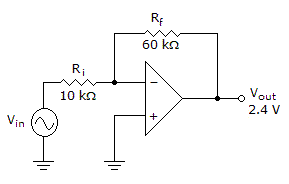# Electronics - Operational Amplifiers

### Exercise :: Operational Amplifiers - Filling the Blanks

11.The input voltage must be ______ in the given circuit to obtain the given output voltage.

 A. 343 mV B. 400 mV C. 480 mV D. 600 mV

Explanation:

No answer description available for this question. Let us discuss.

12.

The voltage required to force the differential output to zero is called _______.

 A. input-offset current B. input-offset voltage C. input bias current D. slew rate voltage

Explanation:

No answer description available for this question. Let us discuss.

13.

If the input to a comparator is a sine wave, the output is a(n) _________.

 A. ramp voltage B. sine wave C. sawtooth wave D. rectangular wave

Explanation:

No answer description available for this question. Let us discuss.

14.

The common-mode rejection ratio (CMMR) is the ratio of _____ to ______.

 A. the differential voltage gain, the common-mode voltage gain B. the differential power gain, the common-mode power gain C. the ideal voltage gain, the actual voltage gain D. signal voltage, noise voltage

Explanation:

No answer description available for this question. Let us discuss.

15.The voltage gain is ______ for the amplifier in the given circuit.

 A. –4 B. –5 C. –6 D. –7